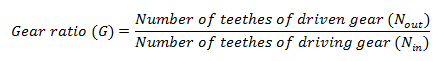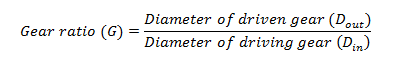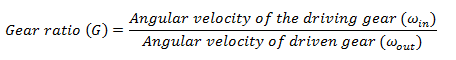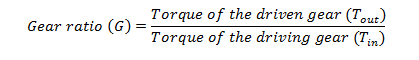# What is Gear Ratio in Transmission System?

## Introduction

“Torque” and “Bhp” we often hear these terms whenever we talk about an automobile vehicle. A car that has to go off-road i.e. 4*4 SUVs, requires good amount of torque and on the other hand the car which has to achieve high speed i.e. supercars, requires good amount of power or speed, Now the questions arise how does a car gives these torque and speed output? How does a car gets different torques and speed whenever required? Let’s find out who is responsible for these.

Gear ratio is the term related to the transmission box of an automobile vehicle which is referred to the ratio of the number of teethes of selected pair of gears (which are in constant mesh) of the output shaft i.e. main shaft, to that of the input shaft i.e. lay shaft, that when selected gives the required torque or speed to the final drive of the vehicle.

When we talk about the manual transmission the gear ratio of the first gear i.e. largest gear of the output shaft and smallest gear of the lay shaft, Gives high torque and the gear ratio of the top gear gives high speed to the final output shaft of the vehicle.

When it comes to automatic or epicyclic transmission, the ratio of the locked annular gear to the planetary and sun gear gives the high torque ratios to the final drive of the vehicle and the ratio of the locked sun gear to the planetary and annular gears gives the high speed ratios to the vehicle.

## Why Do We Need Gear Ratio?Since, we know that the combustion of air-fuel causes the piston movement which in turn rotates the crankshaft of the internal combustion engine which is the engine final output and if we use this output directly as a final output for the wheel rotation than the wheels of the vehicle would rotate at the same torque and speed provided by the crankshaft which is found to be not enough to fulfill the challenges given by the road conditions, And also when it comes to heavy vehicle like a car even 4 cylinder engine cannot provide enough torque that can overcome the vehicle self-weight and cause movement of the vehicle from its initial state.

So we need a set of gear ratios so that-

• The torque and speed provided by the engine can be multiplied, so that the vehicle can face the road challenges.
• In a single drive of a vehicle required variety of torque and speed ratios which engine fails to provide as it can provide limited torque and speed.
• Fuel economy is the main factor as when the load over the engine increases due to its self-weight or other load conditions, Engine tries to combust more and more fuel in order to fulfill the need of the vehicle.
• To reverse the direction of the vehicle, which is not possible with a direct engine output shaft?
• To transmit the power between two different shafts.

## Calculation of Gear Ratio

The gear ratio between the 2 gears in the mesh can be calculated by using following arbitraries of the gears in meshed-

1. Number of teethes (N) – Gear ratio of a pair of gear in mesh is given by the ratio of number of teethes of the driven gear to that of driving gear, Or the ratio of the number of teethes of the output gear to that of input gear.1. Diameter of gears (D)- Gear ratio of the pair of gears in mesh can be calculated by the ratio of the diameter of the driven or output gear to that of the diameter of the driven or input gear.1. Angular velocity (ω) – Gear ratio of the gears in mesh can be calculated by the ratio of the angular velocity of the driving or input gear to that of angular velocity of the driven or output gear.1. Torque(T) – Gear ratio of the gears in mesh can also be calculated by the ratio of the torque of the driven or output gear to that of the torque of the driving or input gear### Example –

Let’s consider a pair of gear A and gear B is in mesh, the gear A is driving and gear B is driven and both the gears have following arbitraries-

Gear A-

Number of teethes- 30

Diameter of gear- 20 cm

Angular velocity- 67cm/sec

Torque- 10 kg-cm

Gear B-

Number of teethes- 40

Diameter of gear- 27 cm

Angular velocity- 50cm/sec

Torque- 13.5 kg-cm

Then the gear ratio G can be calculated using all the arbitraries-

(i) Number of teethes-

Gear ratio (G) = 40/30

= 1.33~1.35

(ii) Diameter of gears-

Gear ratio (G) = 27/20

=1.35

(iii) Angular velocity-

Gear ratio (G) = 67/50

=1.34~1.35

(iv) Torque of gears

Gear ratio = 13.5/10

= 1.35

## Application

The gear ratio is used at the places where the rotation has to transfer between 2 shafts through gears out of which one is driving and the other is driven, The dimensions (number of teethes , diameter of gear ) of the gears is chosen according to the output required.

Some of the applications of the gear ratio are:

• The transmission system of the automobile vehicle in order to get the required torque and speed ratios.
• Sugar cane juice machine for transmitting the high torque required to crush the cane in order to get its juice.
• Lathe machines in order to achieve different torque and speed required to machine different jobs.
• Machines equipped with electric motors as a source of power in order to transmit torque or speed between different parts.